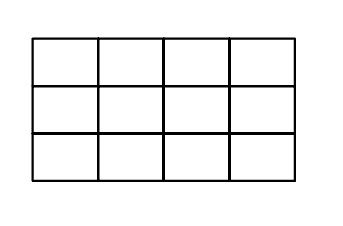# Learning to Count

Counting is hard. It is far more involved than just saying one, two , three. After all, claiming that saying one, two, three means you are counting…is like claiming you can spell just because you know how to say a, b, c .

Sometimes, however, counting does feel as easy as saying one, two, three. Counting these cars, for example, feels pretty darned easy…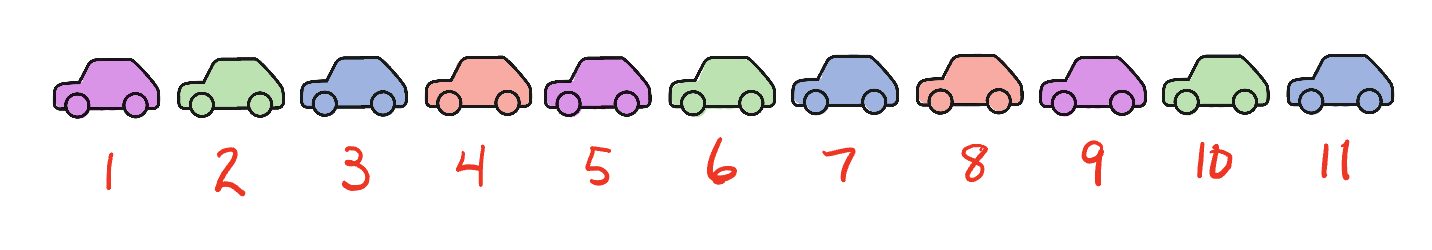But there is more to these cars than just counting them one by one. Perhaps you might notice that the colors follow a predictable pattern that can be used to make the counting easier.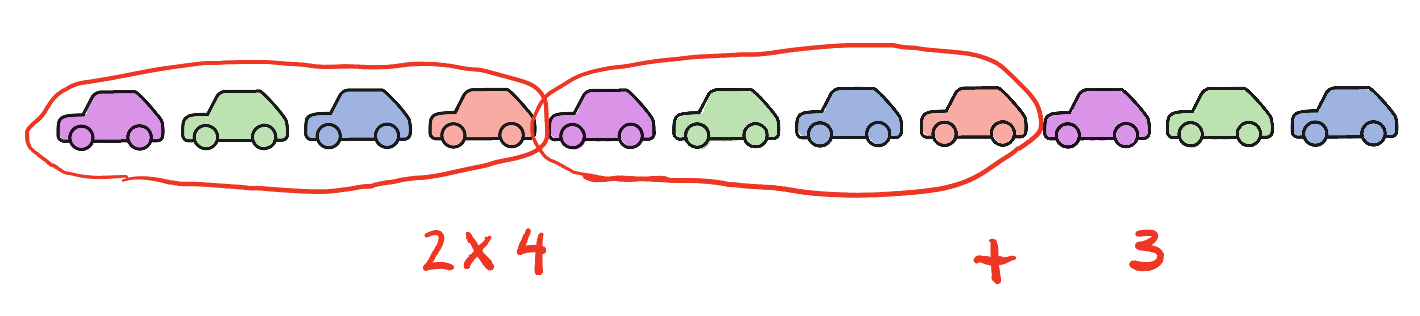How about these dots? How many dots are there?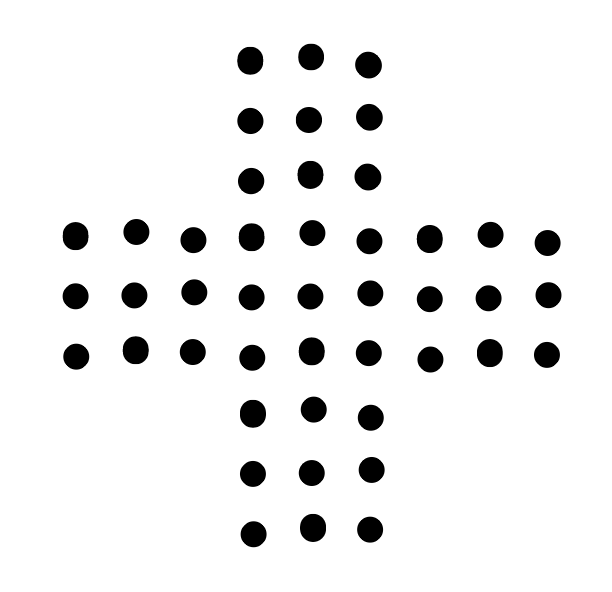If your only tool for counting is to say one, two, three then counting these dots might be tricky…and rife with opportunities for error. But if you group the dots carefully, counting becomes easy.

You might see that the dots can be grouped into five groups of nine dots.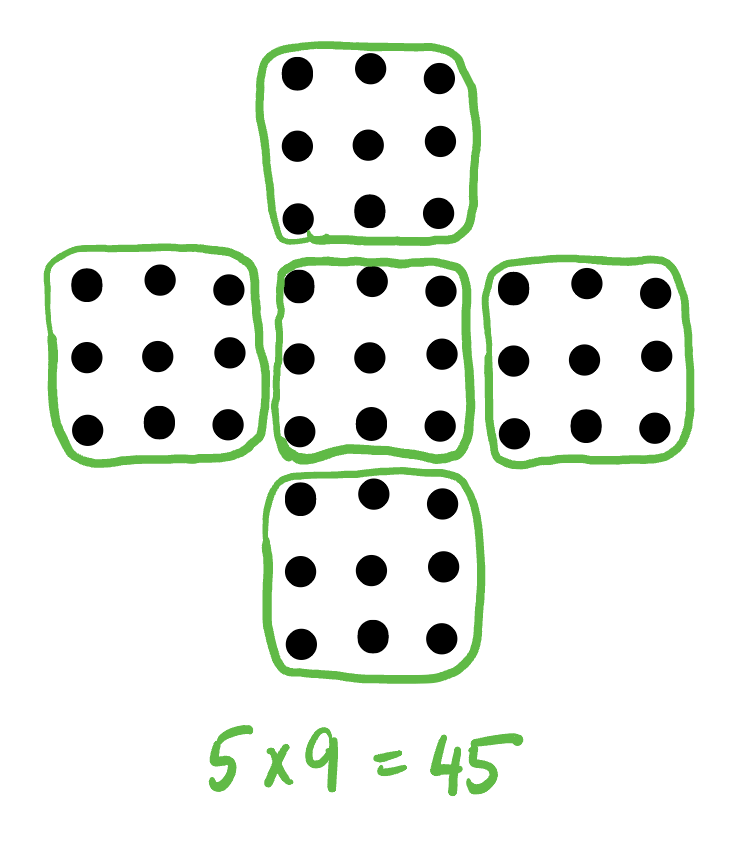Another way to count the dots is to notice the dots can be arranged into groups of 10, with five extra dots in the middle.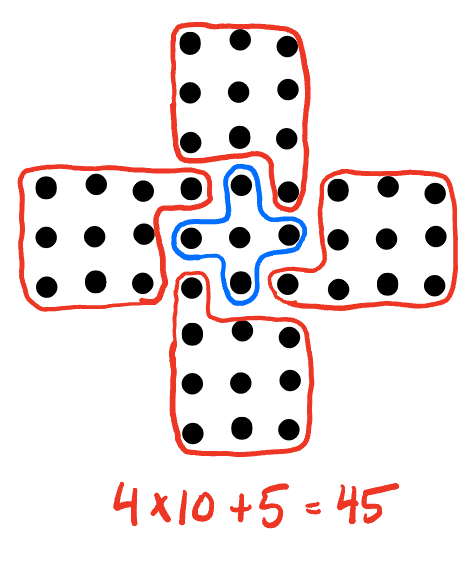So, one way to make counting easier is to look for opportunities to strategically group the objects and let multiplication do the counting for you.

Another way to count is to look for patterns. For example, how many squares of any size are in this 5 by 5 grid?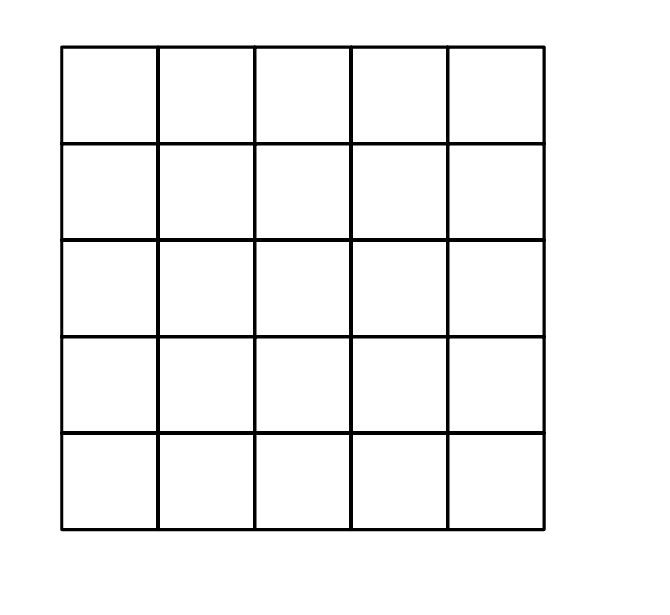The answer is clearly more than just the 25 little one-by-one squares, but how many squares are there?

To find this answer, you might want to try counting them one at a time, but t will get messy real quick. Did I count that one already? I’m not sure. Am I missing one or two? How do I know?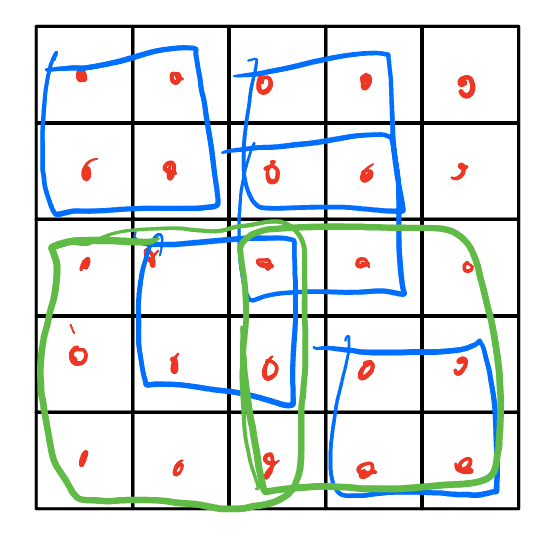So, using a pattern will help with your counting.

Let’s begin by carefully counting the squares one size at a time and recording those numbers in a chart.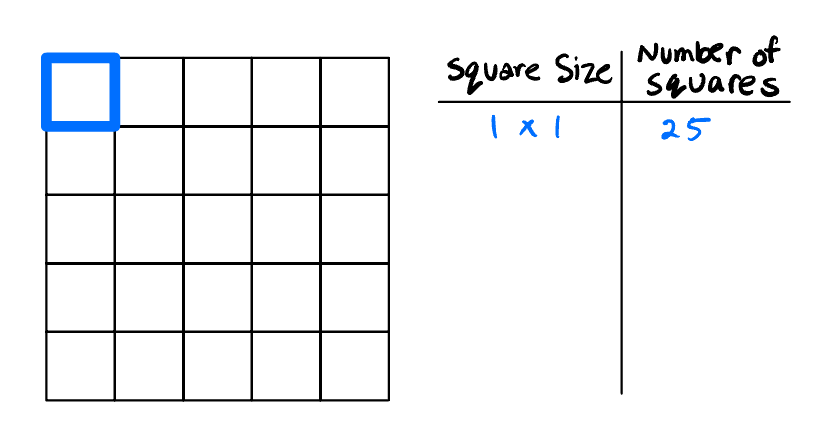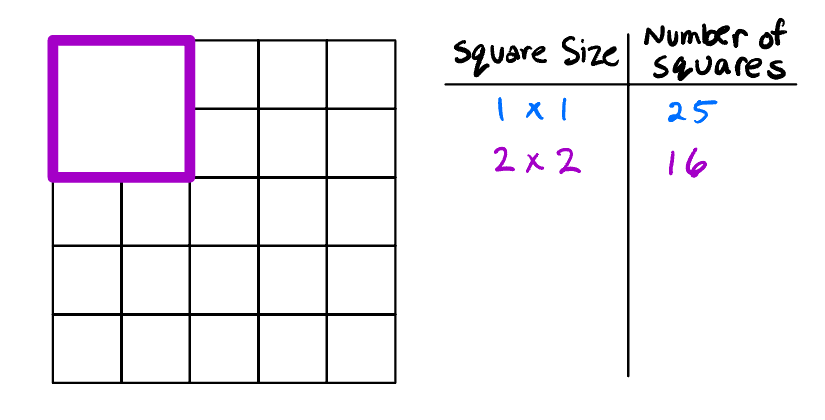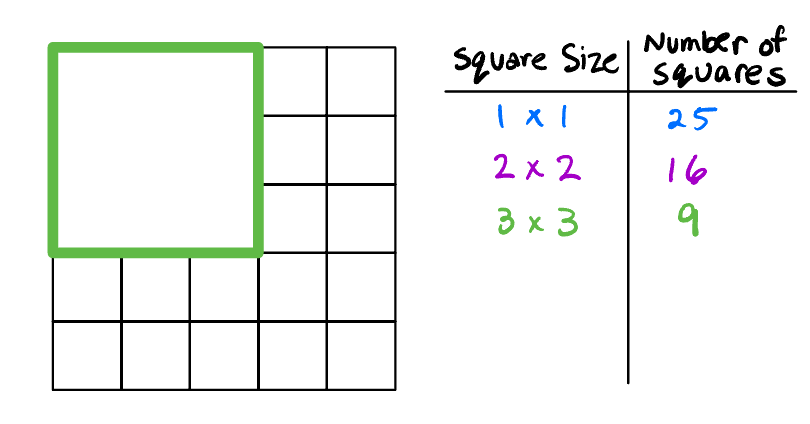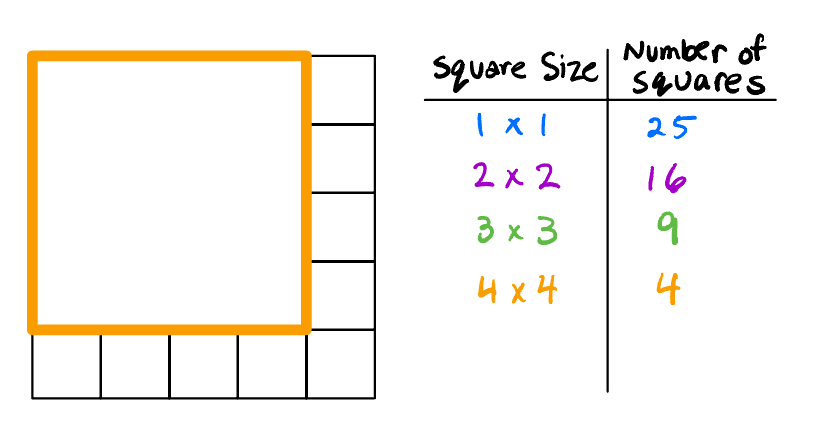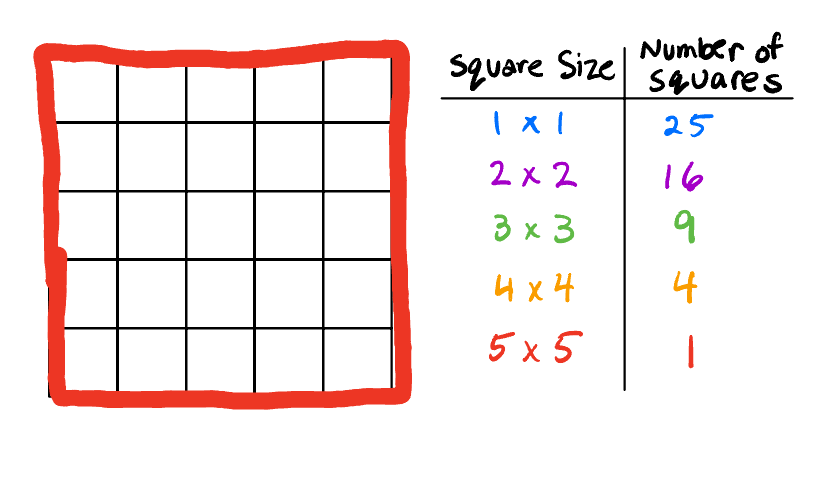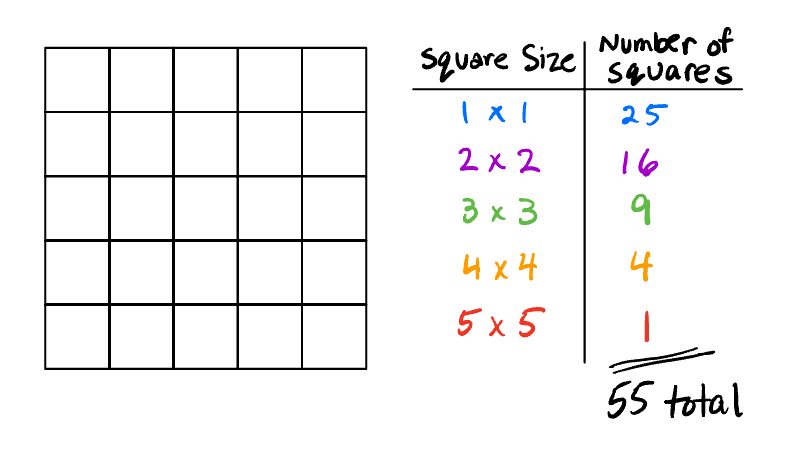How do we know that we counted all the squares? We see a pattern!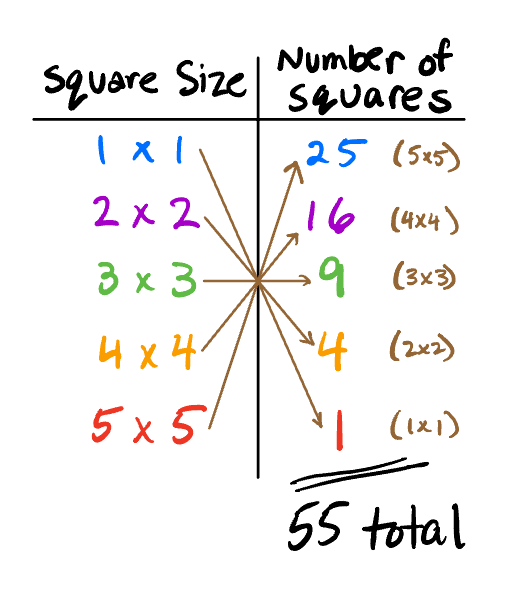Seeing a pattern makes us more certain that we have found all the squares. We can also use the pattern to find the number is squares in larger grids.

Finding the number of squares in a 8×8 grid, it becomes as easy as adding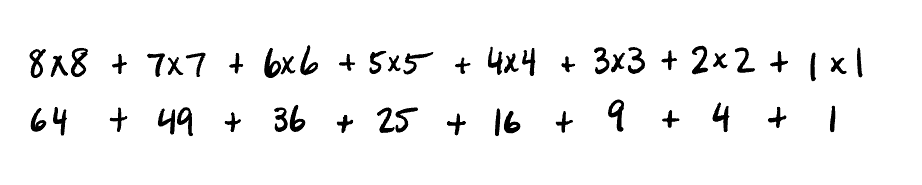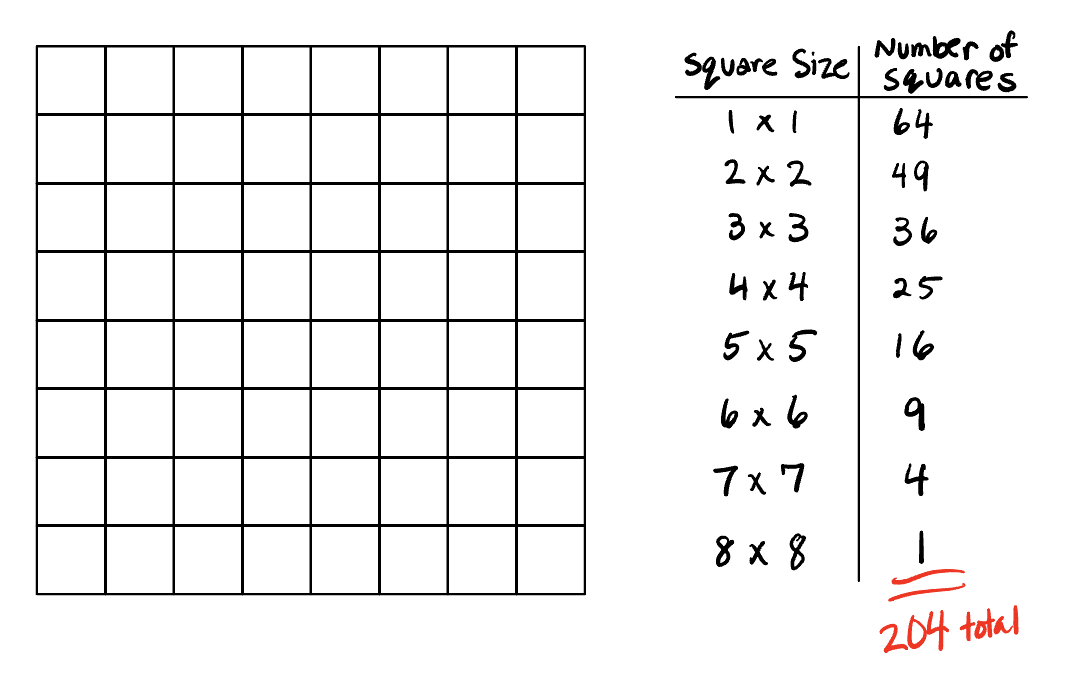# A new way to count.

Let’s go back to the 5×5 grid and count in a different way. We will see a new pattern as a result.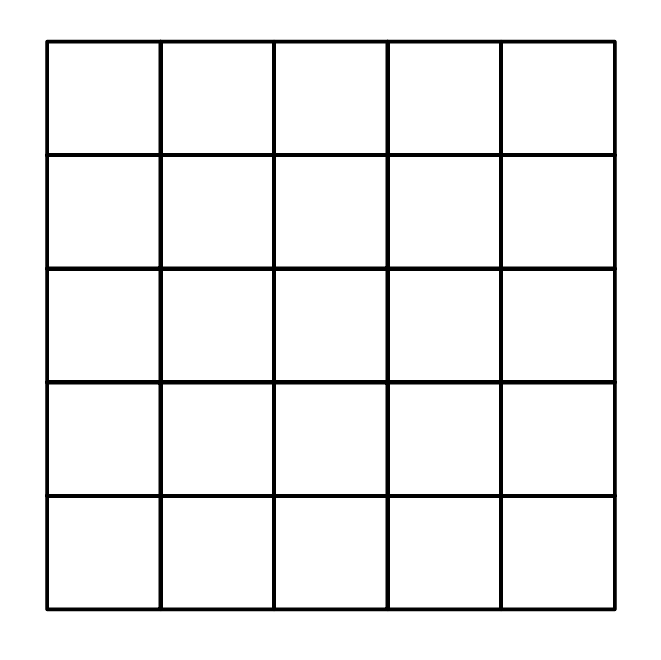We are going to build this grid one square at a time. For each tile added to the figure we will note the number of new squares that are now possible to count.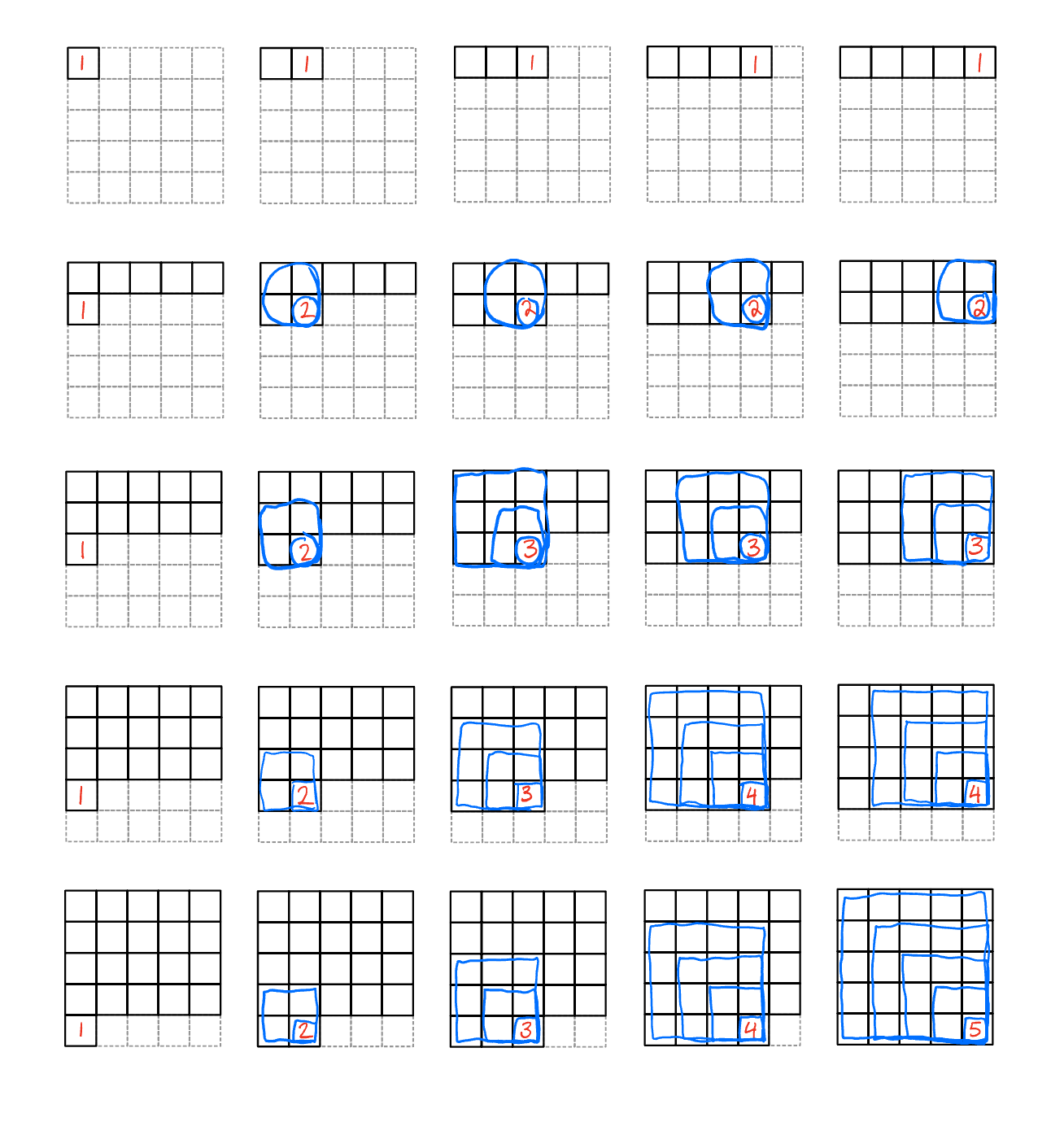Putting all these numbers into a single grid we get…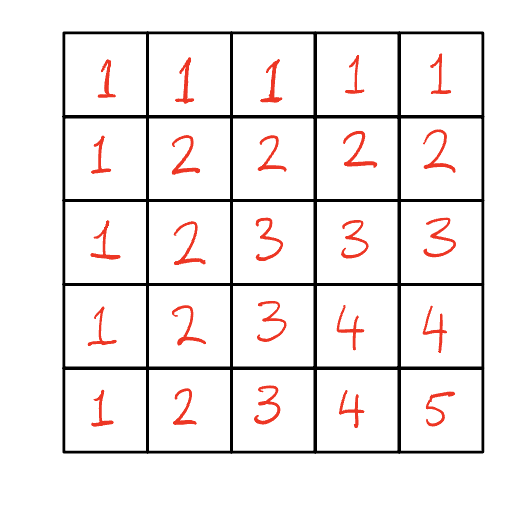We can be sure we’ve found all the squares because there is a symmetry about the numbers.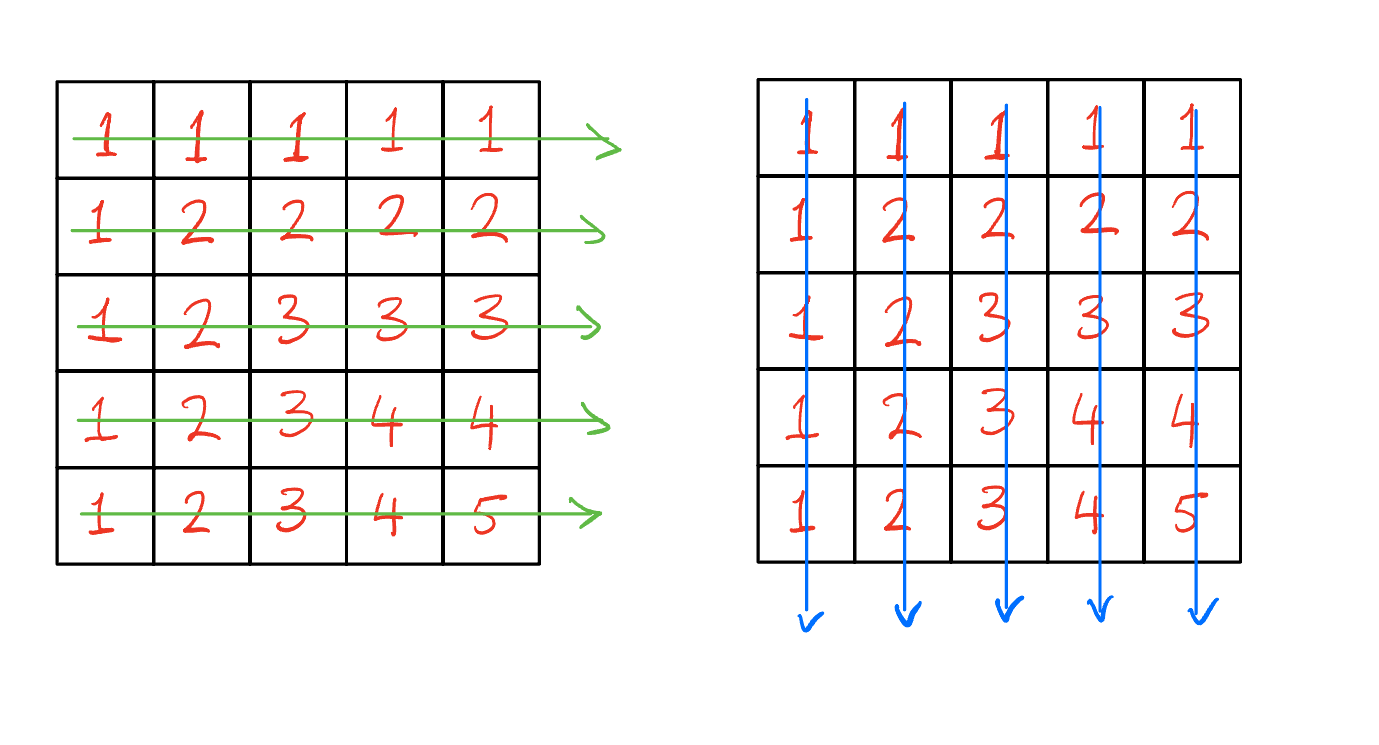Adding up the numbers we get 55 which is the same answer as before.

Let’s use this new style of counting on this figure to count all the triangles.Adding one triangle at a time and recording the number of NEW triangles that are possible with that additional triangle, we get…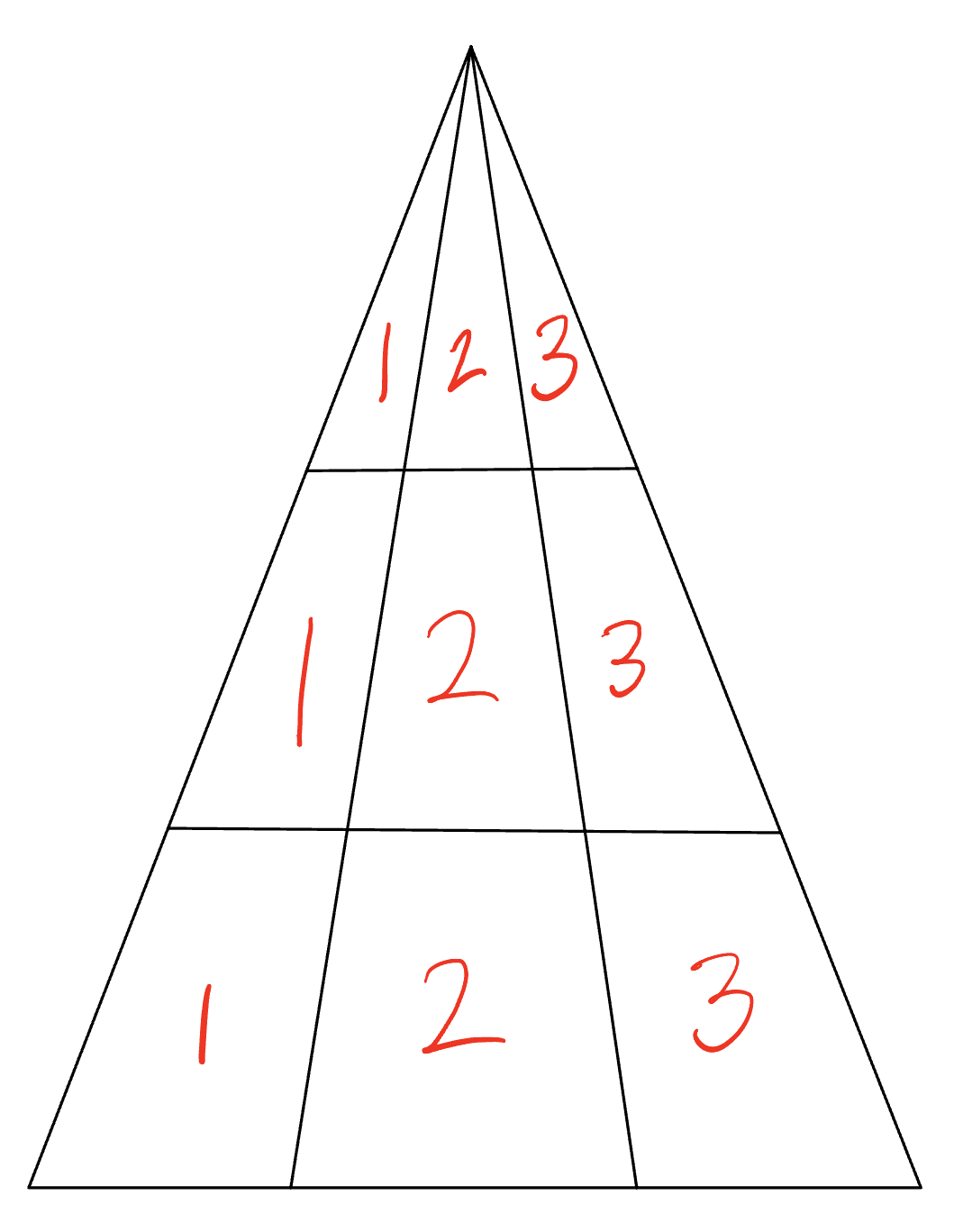It is now pretty easy to see there are 18 triangles in all!

Now it is your turn. Use this new method of counting to count all the rectangles in this figure…